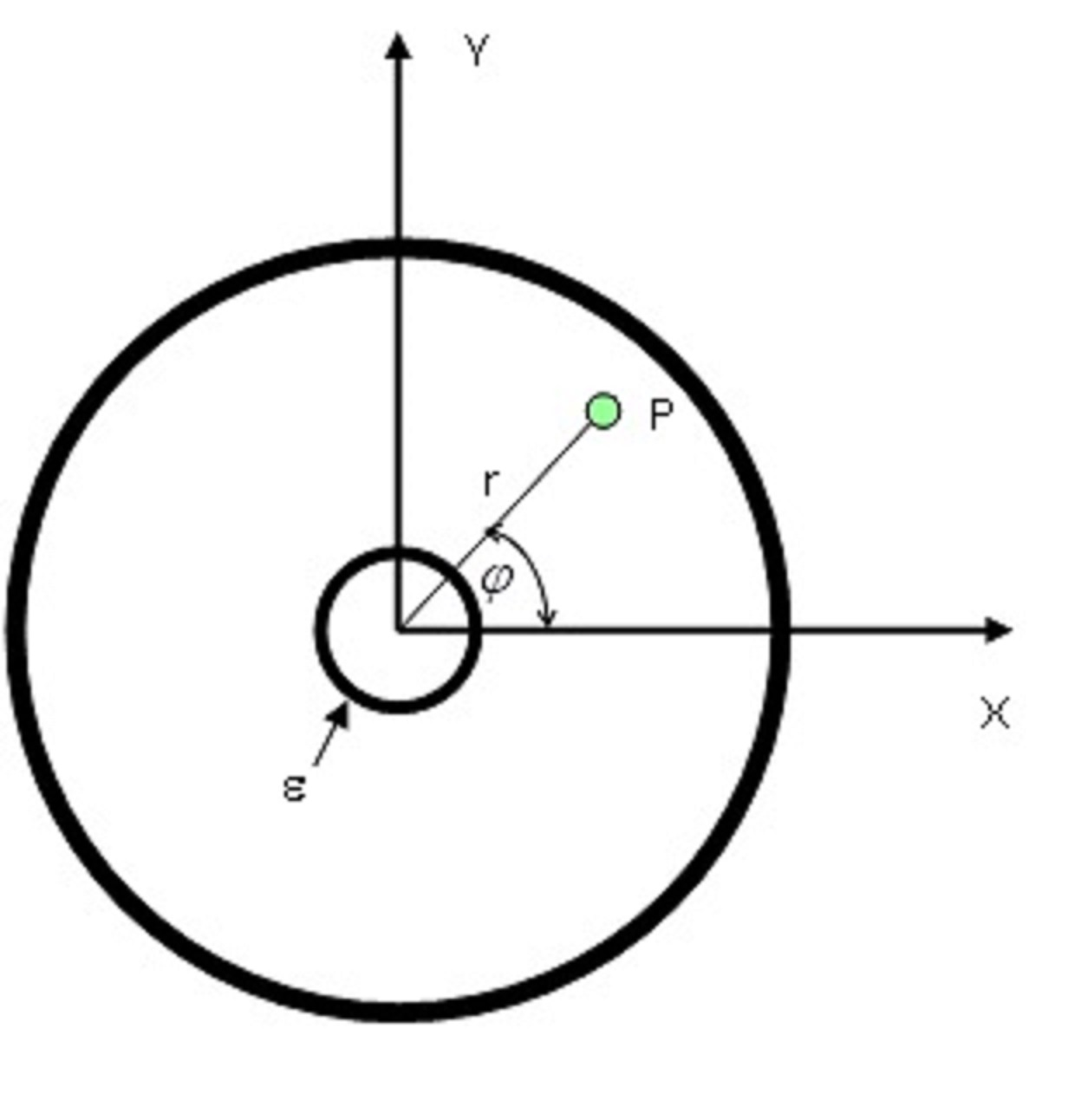# Zernike polynomials in SenSoft

 Zernike polynomials used in Sensoft – our wavefront sensor softwareZernike polynomials represent a particular phase aberration W  at a point P on a circular pupil and they are generally expressed in terms of the normalized radius r of the  pupil and  the  azimuthal  angle φ,  by the following expression:    rn cos (m φ + φ0)

This is the general expression in terms of the Seidel  polynomials. The Standard Zernike polynomials, on the other hand, involve aberration balancing, in which aberrations of a lower order are combined with those of the higher order for reducing the wavefront error. For example, the expression for 3rd order spherical aberration also contains a defocus term.  φ0   is the zero-offset : it gives the orientation of the particular aberration with respect to the x-axis.

Finally, the Annular Zernike polynomials also take into account the effect of the annulus e of the optical element.

SpotOptics wavefront sensor software SenSoft allow you to select among four polynomials to fit to the data

1. Annular Zernike polynomials: Annular Zernike polynomials are based on diffraction theory and take into account the presence of the central obscuration in the pupil. They are the most general form of Zernike polynomials. They are normally used in astronomical wavefront sensors (like Puntino), to take into account the obscuration by the secondary mirror of the telescope
2. Standard Zernike polynomials: Standard Zernike polynomials follow from Annular Zernike polynomials, with the central obscuration factor, and are normalized over the pupil
3. Fringe Zernike polynomials: Fringe Zernike polynomials are the same as Standard Zernike polynomials without the normalization factors. They are mainly used in the field of interferometry (as suggested by the name)
4. Seidel polynomials: The Seidel polynomials are the extension of Seidel aberrations from geometrical optics
 Aberration Tilt (n=1,m=1) Standard Zernike  2r cos(φ + φ0) Annular Zernike 2r cos(φ + φ0) ⁄ √(1 + ε2) Seidel r cos(φ + φ0) Defocus (n=2,m=0) √3 (2 r2 – 1) √3 [2 r2 – (1 + ε2)] /(1 – ε2) r2 Coma (n=3,m=1) √8 (3 r3 – 2r) cos(φ + φ1) √8 [3 (1 + ε2) r3 – 2(1 + ε2 + ε4 )r] cos(φ + φ1) /{(1 – ε2) [ (1 + ε2)√(1 + 4ε2 + ε4 )]} r3 cos(φ + φ1) Spherical 3rd order (n=4,m=0) √5(6 r4 – 6r2 +1) √5[6 r4 – 6(1 + ε2)r2 + (1 + ε2 + ε4 )] /(1 – ε2)2 r4 Astigmatism (n=2,m=2) √6  r2 cos(2φ + φ2) √6  r2 cos(2φ + φ2) / √(1 + ε2 + ε4 ) r2 cos(2φ + φ2) Triangular Coma (n=3,m=3) √8  r3 cos(3φ + φ3) √8  r3 cos(3φ + φ3) / √(1 + ε2 + ε4 + ε6) r3 cos(3φ + φ3) Quadratic Astigmatism (n=4,m=4) √10  r4 cos(4φ + φ4) √10  r4 cos(4φ + φ4) / √(1 + ε2 + ε4 + ε6 + ε8) r4 cos(4φ + φ4)